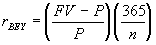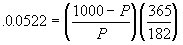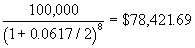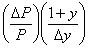Spring 1998

Information for the Midterm

The midterm will have two questions based on the material from Chapters 6, 7 and 8 of Bodie, Kane and Marcus, 3rd. edition.. Here are some things that you should know:

• Equations you should know:
• Chapter 6: 6.1, 6.2, 6.3,
• Chapter 7: 7.1, 7.2, 7.3, 7.7, 7.8, formula in footnote 7, p. 202,
• Chapter 8: 8.1, 8.2, 8.7, definition of beta in no. 6 on p. 261.
• How to compute portfolio weights given the dollar amount invested in each asset in a portfolio.
• How to compute the beta of a portfolio, given the betas of the individual assets making up the portfolio. (Take the weighted average of the individual betas.)
• How to compute the holding period return on an investment.
• How to apply the CAPM formula, eqn. 8.7 to figure out correct stock pricing based on betas.
• How to use the CAPM formula to evaluate portfolios based on their betas.
• The meaning of diversification.

Sample Midterm Exam

Solution: a. The expected return = 12%, because the market has a beta of 1.

The expected return is 4%, because all of the risk of a zero-beta security is diversifiable. Apply the CAPM formula (eqn. 8.7).

Applying eqn. 8.7, we see that the appropriate required return = 4 -0.5(12-4) = 0%. The holding period return = (41-40 +3)/40 = 10%. Hence the stock is underpriced. The correct price would yield an expected return of 0%, which would require the stock to actually experience a capital loss of \$3. In other words, the correct price, based on the stock’s beta = \$44. Then the holding period return = (41-44+3)/44 = 0%

• Problem 16 on p. 264, Chapter 8.

Solution: a. Eqn. 8.7 tells us the average rate of return that an investor can expect is s/he invests in stocks that are priced correctly according to the CAPM. In order to check if an investor was able to find mispriced stocks, we look at the excess of the investor’s actual return over the average return predicted by the CAPM. In this part of problem 16, we don’t have enough data to answer this question.

b. Now, we can compute the required return according to the CAPM (eqn. 8.7) to be 6 + 1.5(14-6) = 18% for investor 1 and 6 + 1(14-6) = 14% for investor 2. According to these benchmarks, investor 1 earned 19-18 = 1% after adjustment for beta-risk compensation, while investor 2 earned an excess of 16-14 = 2%. Hence investor 2 seems to have been able to pick more underpriced stocks, relative to investor 1.

We do know, however, that the CAPM only predicts an average rate of return. In order to see whether an investor’s apparent superior performance in one year actually reflects superior stock-picking skills, we need to track him over time.

Midterm

• The quiz is closed book.
• Explain all your steps; correct answers without explanations may not be given any credit at all.
• Show all your computations and formulae. Make all your assumptions explicit. If your approach is correct, you will get some credit, even if your arithmetic answer is wrong. So concentrate on getting your logic right.
• If you answer a question, I have the discretion to award you some points, even if you are completely wrong. If you don't attempt the question at all, I can give you no points! So attempt every question.

1. You have decided to pick the following securities for your portfolio, partly using information from http://www.stockscreener.com on the Web, and partly using information from your own sources:

 Stock Amount to Invest Std. Dev. of Returns Beta Tucson Electric Power Company 500 20% 0.76 Kaiser Aluminum Corporation 500 35% 0.90 Fannie Mae 1000 30% 1.15 Freddie Mac 1000 40% 1.18 Unisys Corporation 2000 45% 2.17
1. Compute the beta of the portfolio.
2. If the expected return on the market portfolio for the next year were 15%, and the yield on 1-year T-bills were 6%, what would the expected return on your portfolio be according to the CAPM?
3. If you decided to invest all your money equally in Unisys and Freddie Mac alone, and you knew that the correlation between their returns was 0.4, what would be the standard deviation of returns on your portfolio?

2. You have decided to avoid selecting your own stocks. You go to http://www.morningstar.net and find some stock funds that meet your criteria. Since you figure you can afford to be aggressive at this stage in your life, you first look for the most volatile funds. Among these, you search for diversified funds, since you intend to divided your investments between a low-risk Government Securities fund, and your chosen stock fund.

After a lot of soul searching, you pick Pin Oak Aggressive Stock Fund, which has a standard deviation of returns of 33.5%. From the information presented, you glean that the beta of the fund is 1.62, and the R-squared of the regression of fund returns on the S&P 500 Index is 0.44. The 3-year average annual return on this fund is 18.34%, but you think that next year the fund will do much better, and your estimate of the expected return for this fund is 40%. The 3-year average annual return on the S&P 500, in contrast, is 31.5%, which you think is a good indication of what the market is likely to do in the next year. The yield on the Government securities fund that you’ve decided to go with is 7%, which has been the average risk-free rate over the last three years, as well.

1. Can you quantify how well or badly this fund did in terms of individual stock selection over the last three years?
2. If you have a risk-aversion coefficient of 3, how much would you invest in Pin Oak, and how much in the Government Securities Fund?
3. What is the standard deviation of returns on the portfolio that you choose?
4. How confident are you that this fund is really diversified? Explain your answer.

Solutions:

1.

1. The beta of the portfolio is the weighted average of the betas of the individual stocks. The portfolio weights are 10%, 10%, 20%, 20% and 40%. Hence the portfolio beta = 0.1(0.76) + 0.1(0.90) + 0.2(1.15) + 0.2(1.18) + 0.4(2.17) = 1.5
2. The expected return on the portfolio = 6 + 1.5(15-6) = 19.5%
3. In this case, the portfolio weights would be 50% and 50%, and the portfolio variance = (0.5)2(40)2 + (0.5)2(45)2 + 2(0.5)(0.5)(0.4)(40)(45) = 1086.25. The standard deviation = (1086.25)0.5 = 32.96%

2.

1. The expected return on this fund according to the CAPM = 7 + 1.62(31.5 - 7) = 46.69%. The actual average return was 18.34%. Hence, on a risk-adjusted basis, the fund underperformed by 46.69 - 18.34 = 28.35%. It was therefore very bad in its stock selection.
2. With a risk-aversion coefficient of 3, I would invest (40 - 7)/(.01)(3)(33.5)2 = 0.98, i.e. 98% of my money in Pin Oak and 2% in the Government Securities fund.
3. The standard deviation of the portfolio = 0.98(33.5) = 32.84%
4. This fund does not seem to be very diversified, since the R2 is so low. Only 44% of the variance of returns on the fund are explained by market movements.

Final

• The quiz is closed book.
• Explain all your steps; correct answers without explanations may not be given any credit at all.
• Show all your computations and formulae. Make all your assumptions explicit. If your approach is correct, you will get some credit, even if your arithmetic answer is wrong. So concentrate on getting your logic right.
• If you answer a question, I have the discretion to award you some points, even if you are completely wrong. If you don't attempt the question at all, I can give you no points! So attempt every question.

1. Here is an article from the New York Times website for April 22, 1998. The story was filed at 3:05 pm EDT and was entitled, "Bond Prices Rise." The text of the article was as follows:

The price of the Treasury's main 30-year bond was up 1/8 point, or \$1.25 per \$1,000 in face value, around midday, while its yield slipped to 5.94 percent from 5.95 percent late Tuesday. Prices and yields move in opposite directions. Yields on three-month Treasury bills fell to 5.05 percent as the discount lost 0.01 percentage point to 4.93 percent. Six-month yields fell to 5.22 percent as the discount dropped 0.01 percentage point to 5.03 percent. One-year yields held at 5.37 percent as the discount stayed at 5.11 percent. Yields are the interest bonds pay by maturity, while the discount is the interest at which they are sold.

The 30 year T-bond closed on Tuesday, April 21, 1998 at 102 15/32.

a. (5 points) What can you say about the coupon rate on the 30 year T-bond? Give reasons for your answer.

b. (5 points) Suppose the coupon rate on the bond is 6%. How many dollars would you have to pay if you bought the bond at midday? Assume that the bond was issued on 1/1/98.

c. (5 points) As of midday, what was the price of a 3 month T-bill with a face value of \$1m.? T-bill yields (bond-equivalent) are computed using the formula.

d. (10 points) Assuming that the liquidity premium for 6 month T-bills is negligible, what is the market’s forecast of the three month T-bill rate three months from now?

2. Another article in the New York Times of the same day had the following to say regarding bonds sold the previous day by the Ford Motor Credit Corporation:

Strong demand for the Ford Motor Credit securities allowed the company to increase the size of the issue from its initial plans. The amount of five-year notes was raised by \$750 million, to \$2.25 billion. The notes sold at a price to yield 6.17 percent -- about 55 basis points, or hundredths of a percentage point -- more than the rate of a Treasury note with a comparable maturity.

a. (10 points) What is the duration of these bonds? Assume that the bonds sold at par.

b. (10 points) Suppose you need to make a payment of \$100,000 four years from today. If you choose to buy these five-year notes today to ensure that you will have enough to make your payment in four years, how much money must you invest in these notes? Hint: What is the rate at which the value of your portfolio will increase per year?

c. (10 points) If the yield on these bonds go up by 20 basis points tomorrow (20/100ths of a percentage point), by how much will the price of the bond change? Estimate the bond price change using the duration of the bond.

d. (10 points) The convexity of this bond can be computed to be 22.43 years. Estimate the bond price change now, using both duration and convexity. Using this estimate, will you still have enough money to make your payment 4 years from now? If not, what will be the amount of the shortfall?

e. (10 points) Suppose you wanted to immunize your liability perfectly using these 5-year notes and a 5-year zero coupon bond also yielding 6.17%. How much money should you invest in the 5-year zero, and how much in the 5-year notes? Note that the duration of a portfolio of two bonds with the same yield-to-maturity is computed using the following formula:, where Di is the duration of bond i.

3. (25 points) Suppose you bought a just-issued 10-year bond last year, with a coupon rate of 5%, paying annual coupons, and priced to yield 10%. What will the after-tax return on the bond be if the bond is currently priced to yield 8%? Assume that the capital gains tax is half of the ordinary tax rate of 40%.

Solutions:

1. a. The coupon rate must be > 5.94%, since the bond’s selling at a premium.

b. The price at midday was 102 19/32. If the coupon rate is 6%, the semiannual coupon is \$30. Assuming that the bond was issued on 1/1/98, that’s 31+28+31+21 = 111 days from the previous coupon payment date. Hence the accrued coupon = 111/180 x 30 = \$18.5. Hence the dollar amount to be paid = (102.59375)x1000/100 + 18.5 = \$1044.44.

c. The yield on the 3-month T-bill = 5.05%. hence,. Solving, P = \$987,566.14.

d. The yield on the 6 month T-bill = 5.22%; hence. Solving, P = \$974,631.80. Hence, the forecast of the three month rate, three months from now = (987,566.14/974,631.80 - 1) x (365/91) = 5.31%

2. a. The duration of the bond = [(1+.0617/2)/(.0617/2)] {1-(1+.0617/2)-10} = 8.755 half years, or 4.3777 years.

b. If we use the yield-to-maturity on the assets to value the liabilities, we'll need to invest. Strictly speaking, we'd need to know the expected four-year holding period return on five-year bonds, which would mean knowing the liquidity premiums.

c.  We know from part a) that the duration of the bond is 4.3777 years.  Hence,= 4.3777. Solving, D P/P = -(4.3777)(0.002)/1.0617) = -0.0082466 = -0.82466%; if P = \$2.25b., D P = -\$18.5548m. If P = \$1000, D P = -\$8.2466.

d. D P/P = -D*D y + 0.5 (Convexity) (D y)2 = -(4.45/1.0617)(0.002) + (0.5)(22.43)(0.002)2 = -0.008338 or -0.8338%. If P = \$1000, D P = -\$8.34; if P = \$750m., D P = -\$6.253m. If I invested 78,421.69, the new value of my portfolio would be (78421.69)(1-0.008338) = 77,767.81. At the rate of 6.37%, the value of the bonds in 4 years will be (77,761.81)(1.0637)4 = 98,558.08. My shortfall would be 100,000 - 99,558.08 = \$441.92

e. We want to construct a bond portfolio with a duration of 4.45 years. If we invest a % in the coupon bond and (1-a )% in the zero, we have 4.45a + 5(1-a ) = 4. Solving, a = -1.82. Shortsell the zero to the extent of 82% of the portfolio, or (0.82)(78421.69) = \$64,305.79; invest \$142,727.89 in the coupon bond.

3. The price of the bond last year would have been \$692.74; if the yield had not changed, the price today would have been 692.74(1.1) = 762.00 including coupon, and 712.00 excluding the coupon of 5%. With the yield of 8%, however, the price is (on the now 9 year bond) 812.55. Hence the capital gain on which capital gains taxes applies is 812.55 - 712 = \$100.55; ordinary tax is paid on 762 - 692.74 = 69.26. Total taxes paid equal (100.55)x0.2 + 69.26x0.4 = \$47.81. The after-tax return is, therefore, (\$50 + \$812.55 - 692.74 - 47.81)/692.74 = 17.61%.